Modal Analysis of Identified Models

Identify state-space models of systems. Use the models to compute frequency-response functions and modal parameters. This example requires a System Identification Toolbox™ license.

Hammer Excitation

Load a file containing three-input/three-output hammer excitation data sampled at 4 kHz. Use the first ${10}^{4}$ samples for estimation and samples $2×{10}^{4}\text{\hspace{0.17em}}$ to $5×{10}^{4}$ for model quality validation. Specify the sample time as the inverse of the sample rate. Store the data as @iddata objects.

rest = 1:1e4;
rval = 2e4:5e4;
Ts = 1/fs;

Estimation = iddata(YhammerMISO1(rest,:),XhammerMISO1(rest,:),Ts);
Validation = iddata(YhammerMISO1(rval,:),XhammerMISO1(rval,:),Ts,'Tstart',rval(1)*Ts);

Plot the estimation data and the validation data.

plot(Estimation,Validation)
legend(gca,'show')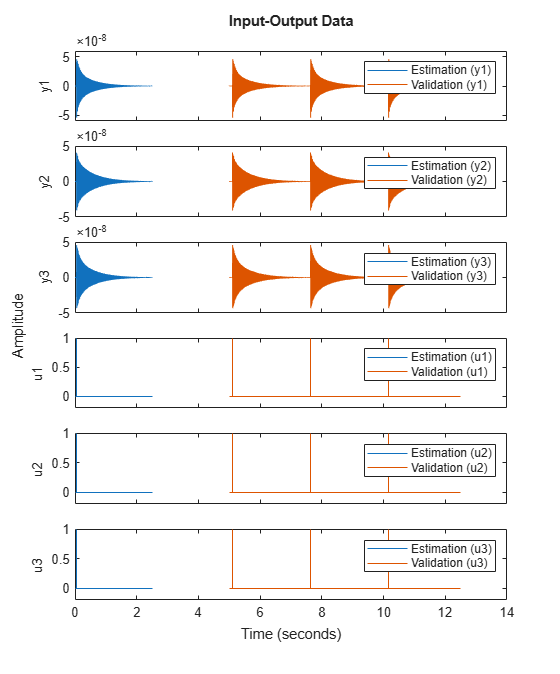Use the ssest function to estimate a 7th-order state-space model of the system that minimizes the simulation error between the measured outputs and the model outputs. Specify that the state-space model has feedthrough.

Orders = 7;
opt = ssestOptions('Focus','simulation');

sys = ssest(Estimation,Orders,'Feedthrough',true,'Ts',Ts,opt);

(To find the model order that gives the best tradeoff between accuracy and complexity, set Orders to 1:15 in the previous code. ssest outputs a log plot of singular values that lets you specify the order interactively. The function also recommends a model order of 7.)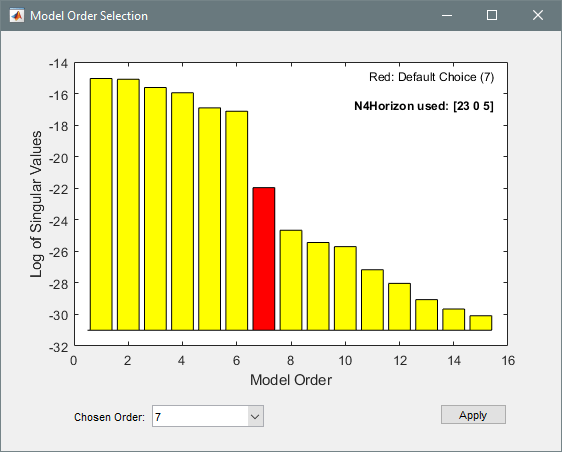Validate the model quality on the validation dataset. Plot the normalized root mean square error (NRMSE) measure of goodness-of-fit. The model describes accurately the output signals of the validation data.

compare(Validation,sys)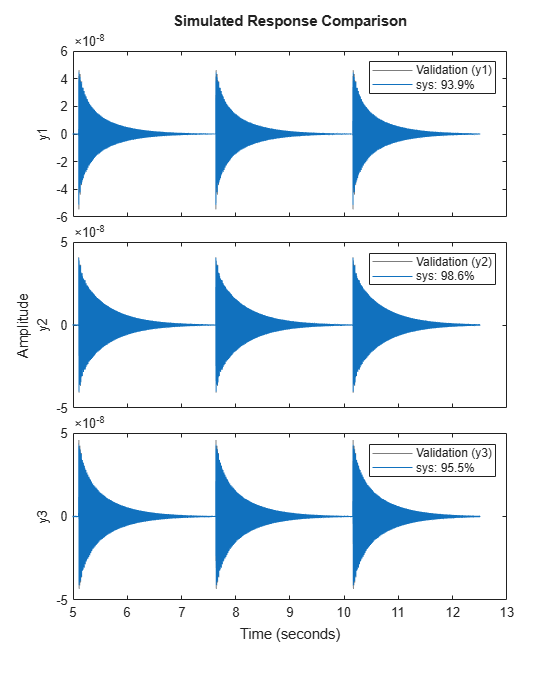Estimate the frequency-response functions of the model. Display the functions using modalfrf without output arguments.

[frf,f] = modalfrf(sys);
modalfrf(sys)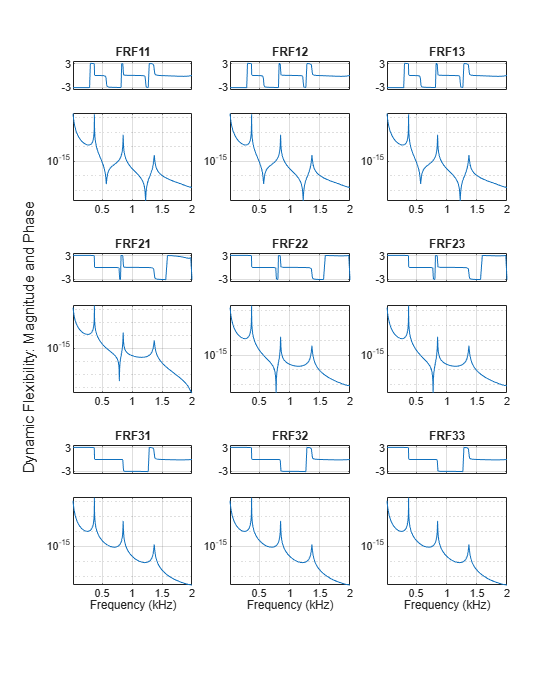Assume that the system is well described using three modes. Compute the natural frequencies, damping ratios, and mode-shape vectors of the three modes.

Modes = 3;
[fn,dr,ms] = modalfit(sys,f,Modes)
fn = 3×1
103 ×

0.3727
0.8525
1.3706

dr = 3×1

0.0008
0.0018
0.0029

ms = 3×3 complex

0.0036 - 0.0019i   0.0039 - 0.0005i   0.0021 + 0.0006i
0.0043 - 0.0023i   0.0010 - 0.0001i  -0.0033 - 0.0010i
0.0040 - 0.0021i  -0.0031 + 0.0004i   0.0011 + 0.0003i

Compute and display the reconstructed frequency-response functions. Express the magnitudes in decibels.

[~,~,~,ofrf] = modalfit(sys,f,Modes);

clf
for ij = 1:3
for ji = 1:3
subplot(3,3,3*(ij-1)+ji)
plot(f/1000,20*log10(abs(ofrf(:,ji,ij))))
axis tight
title(sprintf('In%d -> Out%d',ij,ji))
if ij==3
xlabel('Frequency (kHz)')
end
end
end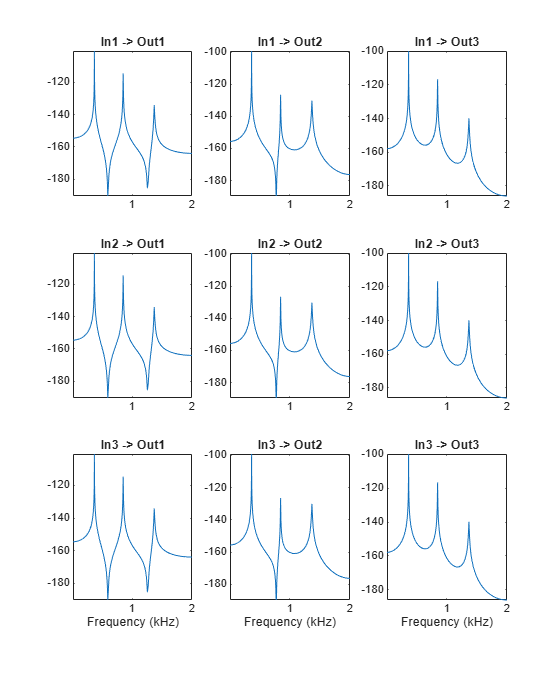Controlled Unstable Process

Load a file containing a high modal density frequency-response measurement. The data corresponds to an unstable process maintained at equilibrium using feedback control. Store the data as an idfrd object for identification. Plot the Bode diagram.

G = idfrd(permute(FRF,[2 3 1]),f,0,'FrequencyUnit','Hz');
figure
bodemag(G)
xlim([0.01,2e3])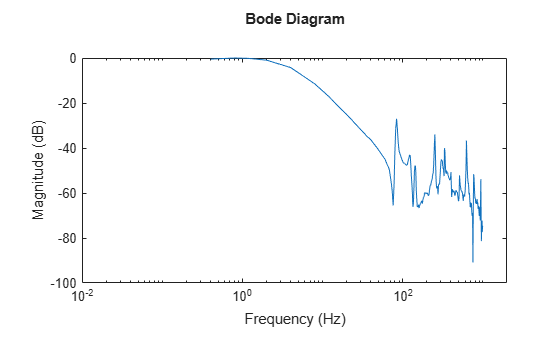Identify a transfer function with 32 poles and 32 zeros.

sys = tfest(G,32,32);

Compare the frequency response of the model with the measured response.

bodemag(G,sys)
xlim([0.01,2e3])
legend(gca,'show')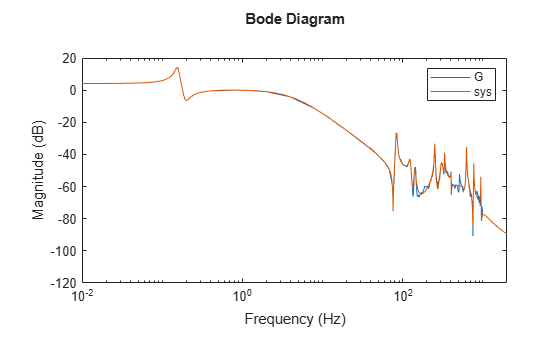Extract the natural frequencies and damping ratios of the first 10 least-damped oscillatory modes. Store the results in a table.

[fn,dr] = modalfit(sys,[],10);
T = table((1:10)',fn,dr,'VariableNames',{'Mode','Frequency','Damping'})
T=10×3 table
Mode    Frequency     Damping
____    _________    _________

1      82.764       0.011304
2      85.013       0.015632
3      124.04       0.025252
4      142.04       0.017687
5      251.46      0.0062182
6      332.79      0.0058266
7      401.21      0.0043645
8      625.14      0.0039247
9      770.49       0.002795
10      943.64      0.0019943